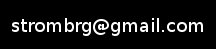treap.py is a python module (more than one actually) for implementing a "treap", which is a datastructure that is a hybrid of a binary heap and a binary tree.

It was originally GPLv3-licensed, but now it's Apache v2 licensed.

This treap module is designed to have a dictionary-like interface:

```\$ python
Python 2.6.4 (r264:75706, Nov  2 2009, 14:38:03)
[GCC 4.4.1] on linux2
>>> import treap
>>> t = treap.treap()
>>> for i in xrange(8):
...     t[i] = 2**i
...
>>> for i in t.keys():
...     print t[i]
...
1
2
4
8
16
32
64
128
>>>
```

However, it's also ordered:

```>>> print list(t)
[0, 1, 2, 3, 4, 5, 6, 7]
>>> print t.find_min()
0
>>> print t.find_max()
7
>>>
```

It comes with a pure python version, and a version that has the more computationally-intensive portion in cython. The two are automatically derived from a single m4-preprocessed file.

Here's a table comparing the asymptotic running time of various python datastructures across a variety of operations. As you can see, like any datastructure it's not stellar at everything, but it does have a nice set of tradeoffs:

 unsorted list (array) sorted list (array) dictionary (hash) minheap treap insert lowest value O(1) O(n) O(1) O(log2(n)) O(log2(n)) insert highest value O(1) O(1) O(1) O(log2(n)) O(log2(n)) insert arbitrary value O(1) O(n) O(1) O(log2(n)) O(log2(n)) remove lowest value O(n) O(n) O(n) O(log2(n)) O(log2(n)) remove highest value O(n) O(1) O(n) O(n) O(log2(n)) remove arbitrary O(n) O(n) O(1) O(n) O(log2(n)) lookup lowest value O(n) O(1) O(n) O(1) O(log2(n)) lookup highest value O(n) O(1) O(n) O(n) O(log2(n)) lookup arbitrary value O(n) O(log2(n)) O(1) O(n) O(log2(n)) sort all values O(n*log2(n)) O(1) (it's already sorted) O(n*log2(n)) O(n*log2(n)) Depending on what you need to do:O(1) It's already sortedO(n) (requires a tree traversal)

If asymtoptic running time is something you don't want to think about, you might just consider that running times can be sorted like the following, for large inputs, where O(1) is fastest and O(n*log2(n)) is slowest:

• O(1)
• O(log2(n))
• O(n)
• O(n*log2(n))

The download also comes with a "nest.py" module, that's intended to make it easy to keep the lowest (or highest) p objects (objects having a __cmp__ method) out of a collection of q objects.

Looking for duptreaps? Check out dupdict_mod.

Hits: 20254
Timestamp: 2022-10-04 19:17:36 PDT

Back to Dan's tech tidbits

You can e-mail the author with questions or comments: